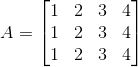Construct a 3×4 matrix A = [ai j] whose elements ai j are given by ai j = j

Asked by Aaryan | 1 year ago |  70

##### Solution :-

Given ai j = j

Let A = [ai j]2×3

So, the elements in a 3×4 matrix are

a11, a12, a13, a14, a21, a22, a23, a24, a31, a32, a33, a34

a11 = 1

a12 = 2

a13 = 3

a14 = 4

a21 = 1

a22 = 2

a23 = 3

a24 = 4

a31 = 1

a32 = 2

a33 = 3

a34 = 4

Substituting these values in matrix A we get,Answered by Sakshi | 1 year ago

### Related Questions

#### Find the values of a, b, c and d from the equations

Find the values of a, b, c and d from the equations:

#### Find x, y, a and b if

Find x, y, a and b if

#### Find x, y, a and b if

Find x, y, a and b if

Construct a 4 × 3 matrix A = [ai j] whose elements ai j are given by $$a_{i j} = \dfrac{(i – j)}{(i + j)}$$